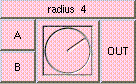RADIUSTHE BOX'S MAIN HELP: This function returns the radius of a circle inscribed in the square defined by A and B. For best results A and B should be perpendicular. Such as u and v or the lengths of two perpendicular vectors. A (input): This must be a scalar. For best results A and B should be perpendicular. Such as u and v or the lengths of two perpendicular vectors. B (input): This must be a scalar. For best results A and B should be perpendicular. Such as u and v or the lengths of two perpendicular vectors. OUT (output): This function returns the radius of a circle inscribed in the square defined by A and B. For best results A and B should be perpendicular. Such as u and v or the lengths of two perpendicular vectors.

<-- BACK TO Math